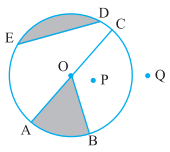### Chapter - 4 Basic Geometrical Ideas

Q
##### Basic Geometrical Ideas

Question:

1. From the figure, identify :

(a) the centre of circle           (b) three radii

(c) a diameter                        (d) a chord

(e) two points in the interior   (f) a point in the exterior

(g) a sector                            (h) a segmentSolution: (a) O is the centre of the circle.

(b) OA, OB and OC are the radii of the given circle.

(c) AC is the diameter of the circle.

(d) ED is the chord in the circle.

(e) O and P are the points in the interior of the circle.

(f) Q is the point in the exterior of the circle.

(g) AOB is the sector in the circle.

(h) ED is the segment in the circle.

2. (a) Is every diameter of a circle also a chord?

(b) Is every chord of a circle also a diameter?

Solution: (a) Yes, in a circle the diameter is the longest chord of the circle.

(b) No, every chord is not a diameter.

3. Draw any circle and mark

(a) its centre                     (b) a radius

(c) a diameter                   (d) a sector

(e) a segment                   (f) a point in its interior

(g) a point in its exterior   (h) an arc

Solution:(a) O is the centre.

(b) OA is the radius.

(c) AB is the diameter.

(d) BOD is a sector.

(e) CA is the segment.

(f) O is the point in the interior.

(g) E is the point in the exterior.

(h)  is the arc.

4. Say true or false :

(a) Two diameters of a circle will necessarily intersect.

(b) The centre of a circle is always in its interior.

Solution: (a) True, two diameters of a circle always intersect at the centre of the circle.

(b) True, the centre of a circle always lie inside the circle.